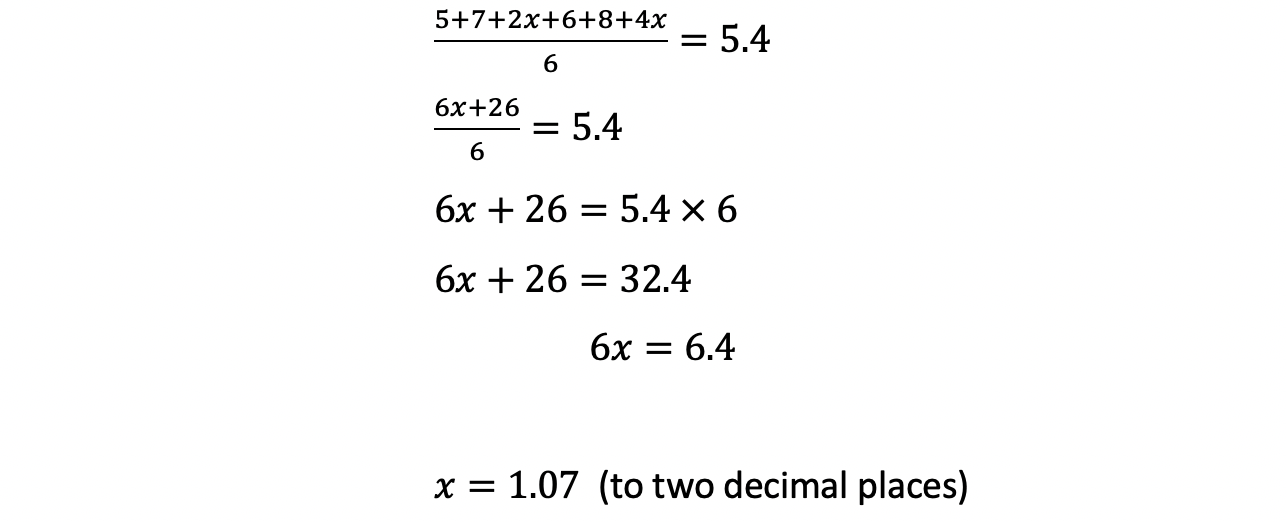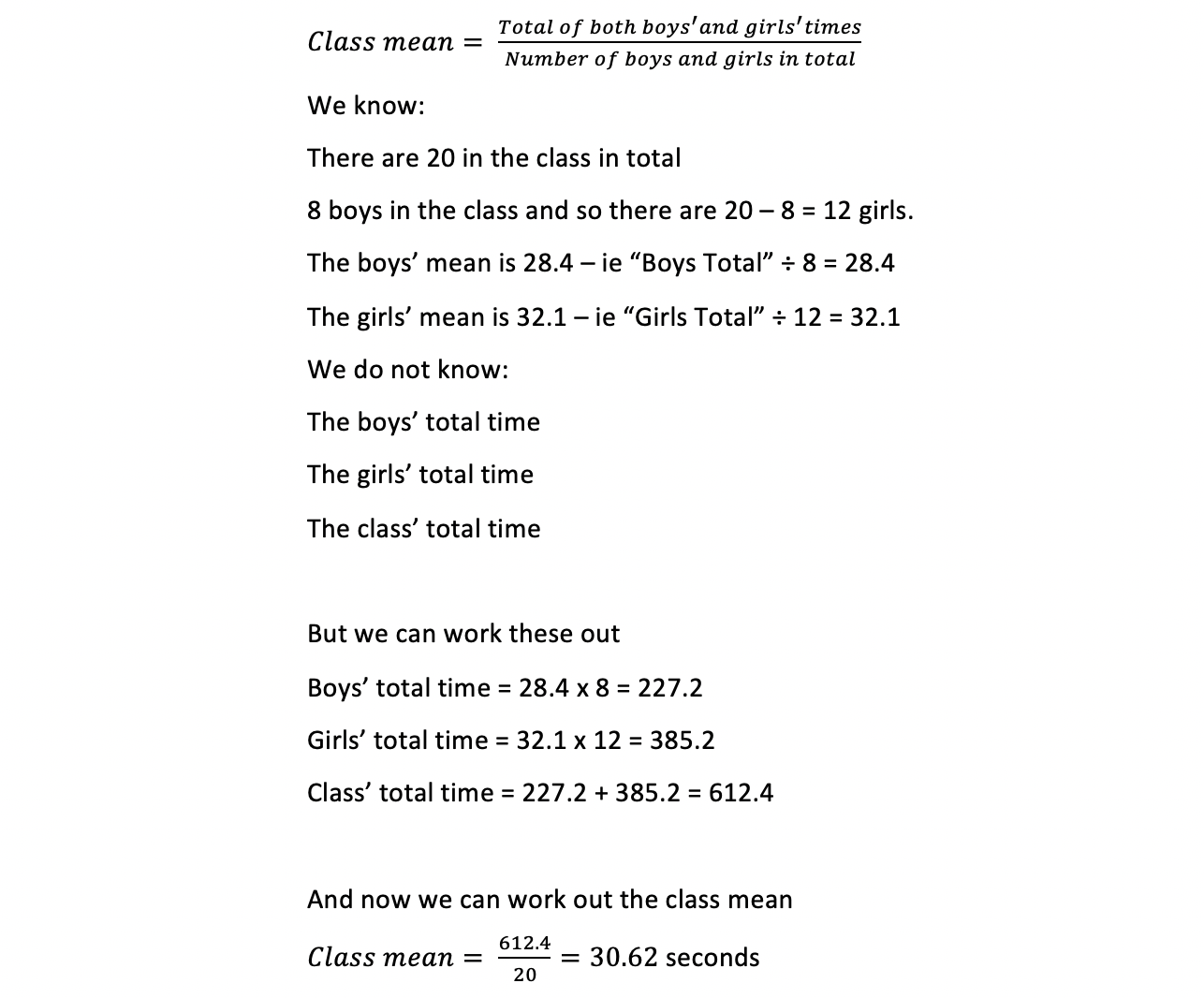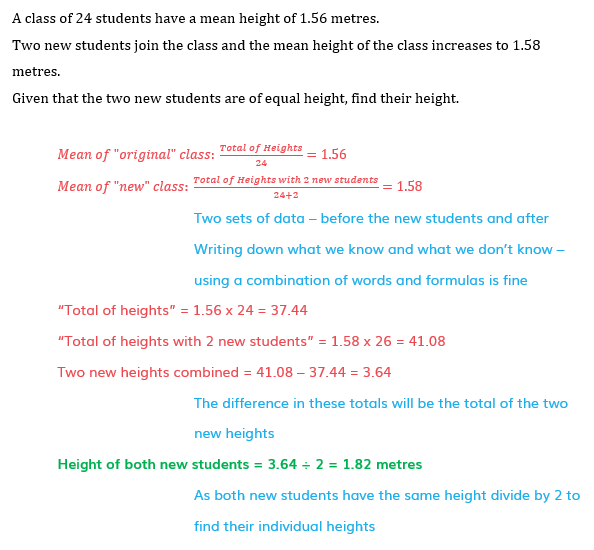# Edexcel IGCSE Maths 复习笔记 6.1.4 Calculations with the Mean

Edexcel IGCSE Maths 复习笔记 6.1.4 Calculations with the Mean

#### Solving problems involving the mean

• Because the mean has a formula it means you could be asked questions that use this formula backwards and in other ways
• Since Mean = Total of values ÷ Number of values then it is a formula involving 3 quantities
• Therefore, if you know 2 of these you can find the other one

#### What may I be asked to do?

• Typical questions ask you to work backwards from a known mean or to combine means for two data sets
• But as this is in the area of problem-solving there may be something unusual that you haven’t seen before so you will need to make sure you understand what the mean is, how it works and what it shows

1. Working backwards

• For example, the mean of the six data values 5, 7, 2x, 6, 8 and 4x have a mean of 5.4Find the value of x
• This is a matter of setting up an equation in x and solving it2. Combining two sets of data

• These sorts of questions are generally harder and need more thinking about
• For example, a class of 20 ran a 100m raceThe mean time for the 8 boys in the class was 28.4 secondsThe mean time for the girls was 32.1 seconds

Find the mean time for the whole class#### Exam Tip

You have used the mean so often in mathematics that you do not normally think of it as a formula. But it is. And as with other work in using formulas you should write down the information you do know, and the information you are trying to find.

#### Worked Example转载自savemyexam